# How to Convert a Decimal Number into Negative Base: A Tutorial

Knoji reviews products and up-and-coming brands we think you'll love. In certain cases, we may receive a commission from brands mentioned in our guides. Learn more.
This article describes how to convert a decimal number into a negative base with a number of illustrations. The process involves four steps: 1. Convert the decimal number into the base b, 2. Break up the number in even and odd parts 3. Convert the odd

The number system we use every day is called the decimal number system, because numbers are represented in base 10 in it. Most of us also know that computers do not use decimal numbers; it uses binary number system, in which the number 2 is used as the base, for numerical calculation as well as storing data. So, is it possible to represent numbers in any other base? And the answer is yes! Any positive integral number (other than 1 and 0) can be used for the purpose. But the most interesting fact is that base of a number can be negative integer, fractional and even imaginary. In this article, I will discuss conversion of a number into negative base and the arithmetic of it.

Number Representation:

Any number “N” represented in base “b” can be expressed as a power expansion of “b” as shown below.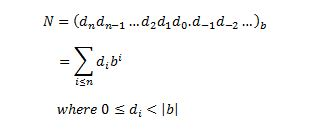One should note that the “point” here should be called a “radix point”, rather than a decimal point.

Method 1:

This method is quite similar to the conversion of decimal to binary. We need to use division algorithm repeatedly such that the remainder is positive in each step and continue until the quotient becomes zero or positive less than mod(b). Then simply assemble the last quotient and remainders in reverse order to obtain the converted number. Here I show two examples, one in base -10 and the other in base -8.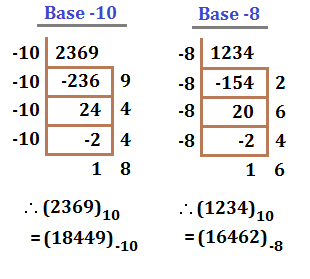Method 2:

The above method works very well for integral numbers but it becomes little difficult for the case of fractional numbers. So, for fractional numbers we shall follow a different treatment.

Suppose we want to convert a decimal number into base (-b). Then, first we shall convert the original number into base b and then transform it into negative base. Here I will assume that readers are already familiar with the conversion process from decimal to other positive integral bases; if not, any standard textbook can be consulted with.

Before we proceed, I want to follow a convention: the positions of a number which have even power in its expansion will be called even places and the rest will be called odd positions. So, the first step is to recognize the even and odd positions and break up the number into even and odd parts.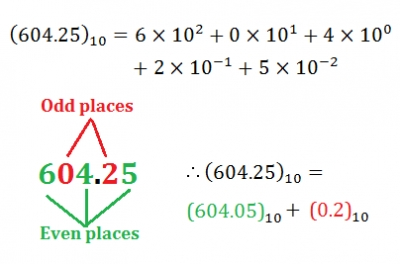The property of even places is that base conversion has no effect for those positional values and for odd places we just need to take complement of the positional values corresponding to the base of the number and replace the left 0 by 1. These are illustrated by the following examples.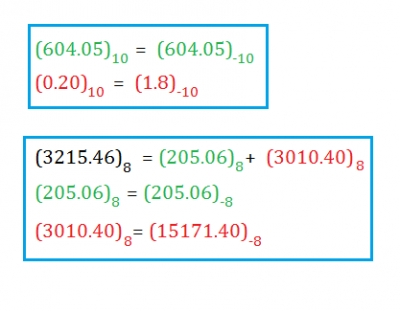As we see even parts undergo no change in the base conversion. I will only explain how the conversion of the odd part for the base 8 number takes place.

a)      Primarily the number is 3010.40; the rightmost zero has no importance

b)      8’s complement of 4 is 4 and the 0 situated at the immediate left is replaced by 1 to get 14

c)       Next 8’s complement of 1 is 7 and the left 0 is replaced by 1 to obtain 17

d)      Finally in the same way from 3 we get 15

e)      Thus in base -8 the number becomes 15171.40

So far we have done the conversion of the individual parts, now we need to combine them, i.e. add those two parts to get the final answer. Addition in negative base is little tricky, because any carry has to be deducted from the left digit rather than adding which is the case for positive base. The results are shown below.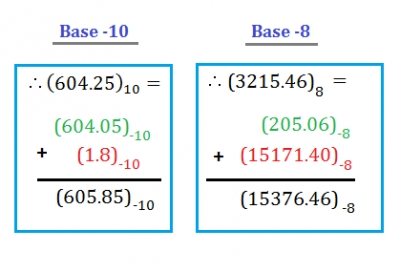As you can check 3215.46 in base 8 has a decimal value of 1677.59375. But in this case, no carry over term has occurred. The following example in base 2 shows the negative carry over case.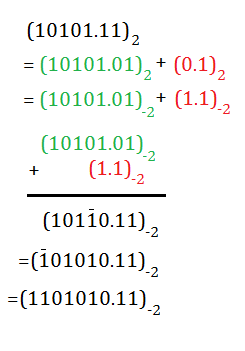Hence, the whole process can be summarized in the following four steps:

1. Convert the decimal number into the base b
2. Break up the number in even and odd parts
3. Convert the odd part into negative base